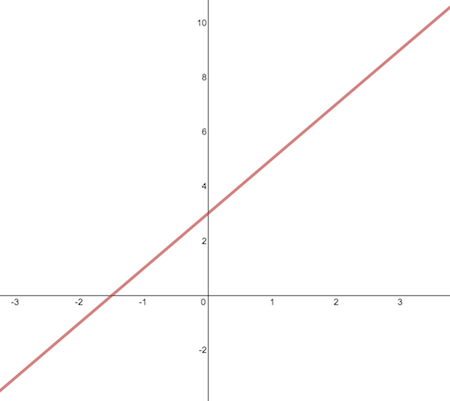Calculus

# Slope of a CurveSally drew the line $$y=2x+3$$ on the board. What is the slope of this line?

What is the slope of the line tangent to the curve $$y = \ln x + 20x$$ at $$x = \frac{1}{7}$$ ?

What is the slope of the line tangent to the graph of the function $$f(x) = - \frac{4}{x}$$ at $$x = \frac{1}{9}$$ ?

What is the slope of the line tangent to the graph of the function $$f(x) = 6 \cos x + 6 \sin x$$ at $$x = 20 \pi$$ ?

What is the slope of the tangent line of the curve $$f(x) = x^2 + 2013$$ at $$x = 29$$?

×